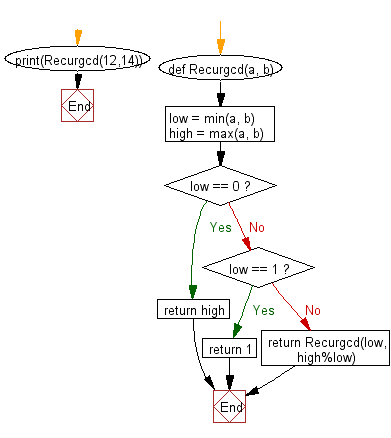﻿ Python Data Structures and Algorithms - gcd of two integers - w3resource# Python Data Structures and Algorithms - Recursion: gcd of two integers

## Python Recursion: Exercise-11 with Solution

Write a Python program to find the greatest common divisor (gcd) of two integers.

Sample Solution:-

Python Code:

``````def Recurgcd(a, b):
low = min(a, b)
high = max(a, b)

if low == 0:
return high
elif low == 1:
return 1
else:
return Recurgcd(low, high%low)
print(Recurgcd(12,14))
```
```

Sample Output:

```2
```

Flowchart:## Visualize Python code execution:

The following tool visualize what the computer is doing step-by-step as it executes the said program:

Python Code Editor:

What is the difficulty level of this exercise?

Test your Programming skills with w3resource's quiz.

﻿

## Python: Tips of the Day

Decapitalizes the first letter of a string:

Example:

```def tips_decapitalize(s, upper_rest=False):
return s[:1].lower() + (s[1:].upper() if upper_rest else s[1:])
print(tips_decapitalize('PythonTips'))
print(tips_decapitalize('PythonTips', True))
```

Output:

```pythonTips
pYTHONTIPS
```